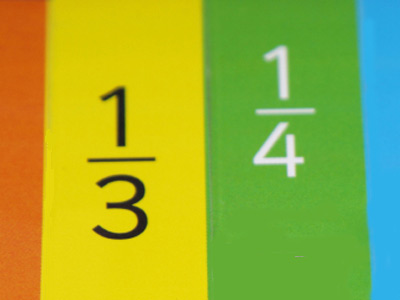Do you know how to write fractions like ¼ as decimals?

# Decimal Numbers 2 (Medium)

Decimal numbers are just another way of writing fractions. For example, one-half can be written as ½, but in decimal, it is 0.5 (that’s because 1 ÷ 2 = 0.5). Familiarity with both forms is essential for the Eleven Plus maths exam.

In this, the second of our medium level quizzes on decimal numbers, your ability to convert decimals to fractions, and vice versa, will be tested to the max! Keep playing until you are fully confident and then try our difficult quizzes on the subject. Before long decimals will cause you no doubt at all!

Make sure to read the comments after you've answered the questions. They are full of helpful tips and explanations that make life much easier.

So, if you are ready, it’s time to begin. Have fun!

1.
If you divide 221 by 1,000, what number do you get?
0.0221
22.1
2.21
0.221
If you divide by 1,000, move the digits 3 places to the right. The decimal point in whole numbers cannot be seen, but it can be imagined as coming straight after the number, followed by zeros (i.e. 221 = 221.000)
2.
What is 0.75 as a fraction?
34
23
45
35
75 is 3 x 25, and we know that 25 is one-quarter of 100. So, 0.75 is three-quarters of 1.
3.
What is ‘one-fifth’ as a decimal?
0.12
0.2
0.25
0.125
100 ÷ 5 = 20 so 1 ÷ 5 = 0.2
4.
What is 0.333 as a fraction?
27
14
13
25
13 as a decimal is 0.33333 - the 3s go on forever. We call this 'recurring'.
5.
What is 0.125 as a fraction?
16
17
18
19
100 ÷ 8 = 12.5. and 12.5 ÷ 100 = 0.125
6.
What is ‘three-eighths’ as a decimal?
0.375
0.333
0.425
0.412
To work this out, first divide 100 by 8 to get 12.5. Next multiply 12.5 by 3 to get 37.5. Finally, divide 37.5 by 100 to get the answer 0.375
7.
If you turn ‘one-fifth’ into a decimal and then multiply by 10, what is the answer?
25
2
200
20
1 ÷ 5 = 0.2 and 0.2 x 10 = 2
8.
What is ‘one-hundredth’ as a decimal?
0.1
0.01
0.001
0.0001
The columns after the decimal point are tenths, hundredths, thousandths, ten-thousandths etc.
9.
If you multiply 0.024 by 1,000, what number do you get?
240
0.24
2.4
24
If you multiply by 1,000, move the digits 3 places to the left
10.
What is 0.666 as a fraction?
45
34
23
56
23 as a decimal is 0.666 recurring (that means the 6s go on forever!).
Author:  Frank Evans